# VB.Net Data Types and Variable Declaration with DIM

## What are Data Types?

Data types determine the type of data that any variable can store. Variables belonging to different data types are allocated different amounts of space in the memory. There are various data types in VB.NET. They include:

• Boolean: the allocated storage depends on the platform of implementation. Its value can be either True or False.
• Byte: allocated storage space of 1 byte. Values range from 0 to 255 (unsigned).
• Char: allocated a space of 2 bytes. Values range from 0 to 65535 (unsigned).
• Date: allocated storage space of 8 bytes. Values range from 0:00:00 (midnight) January 1, 0001 to 11:59:59 PM of December 31, 9999.
• Integer: has a storage space of 4 bytes. Values range between -2,147,483,648 to 2,147,483,647 (signed).
• Long: has a storage space of 8 bytes. Numbers range from -9,223,372,036,854,775,808 to 9,223,372,036,854,775,807(signed).
• String: The storage space allocated depends on the platform of implementation. Values range from 0 to about 2 billion Unicode characters.

In this tutorial, you will learn:

## Type Conversion Functions

There are functions that we can use to convert from one data type to another. They include:

• CBool (expression): converts the expression to a Boolean data type.
• CDate(expression): converts the expression to a Date data type.
• CDbl(expression): converts the expression to a Double data type.
• CByte (expression): converts the expression to a byte data type.
• CChar(expression): converts the expression to a Char data type.
• CLng(expression): converts the expression to a Long data type.
• CDec(expression): converts the expression to a Decimal data type.
• CInt(expression): converts the expression to an Integer data type.
• CObj(expression): converts the expression to an Object data type.
• CStr(expression): converts the expression to a String data type.
• CSByte(expression): converts the expression to a Byte data type.
• CShort(expression): converts the expression to a Short data type.

## Variable Declaration

In VB.NET, the declaration of a variable involves giving the variable a name and defining the data type to which it belongs. We use the following syntax:

```Dim Variable_Name as Data_Type
```

In the above syntax, Variable_Name is the variable name while Data_Type is the name to which the variable belongs.

Here is an example of a valid variable declaration in VB.NET:

```Dim x As Integer
```

In the above example, ‘x’ is the variable name while Integer is the data type to which variable x belongs.

## Variable Initialization

Initializing a variable means assigning a value to the variable. The following example demonstrates this:

```Dim x As Integer
x = 10
```

Above, we have declared an integer variable named ‘x’ and assigned it a value of 10. Here is another example:

```Dim name As String
name = "John"
```

Above, we have declared a string variable name and assigned it a value of John.

If you declare a Boolean variable, its value must be either True or false. For example:

```Dim checker As Boolean
checker = True
```

Above, we have defined a Boolean variable named checker and assigned it a value of True.

Let us demonstrate how to declare and initialize a variable using a code example:

Step 1) Create a New Project

1. Open Visual Studio and click the File menu, Choose New then Project from the toolbar.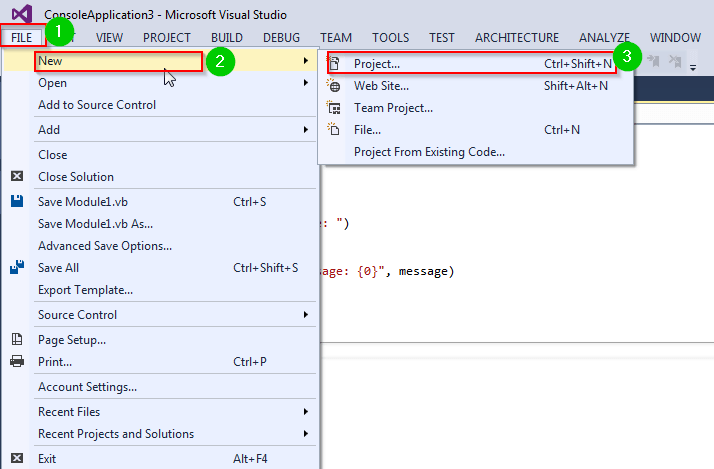On the new window, click Visual Basic from the left vertical navigation pane. Choose Window Forms Application.1. Give it a name and click the OK button. The Project will be created.

Step 2) Create a Button

1. To create a Button, begin by opening the design tab.1. Drag the Button control from the toolbox into the WindowForm: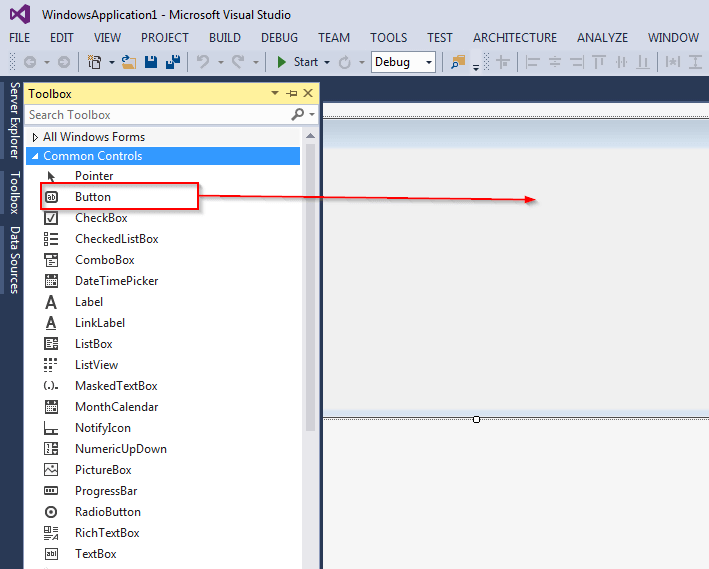Step 3) Click the other tab located to the left of the design tab. You can also double click the button that you have added to the form.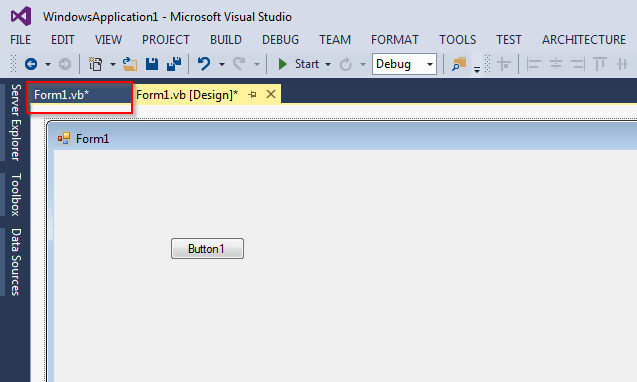Step 4) Add the following code to add text to the control:

```Private Sub Button1_Click(sender As Object, e As EventArgs) Handles Button1.Click

Dim checker As Boolean
checker = True
MsgBox("The value of variable checker is  : " & checker)

Dim x As Integer
x = 32
MsgBox("The value of variable x is  : " & x)

Dim name As String
name = " Guru99 "
MsgBox("The value of variable name is  : " & name)

End Sub
```

Step 5) You can now run the code by clicking the Start button located at the top bar: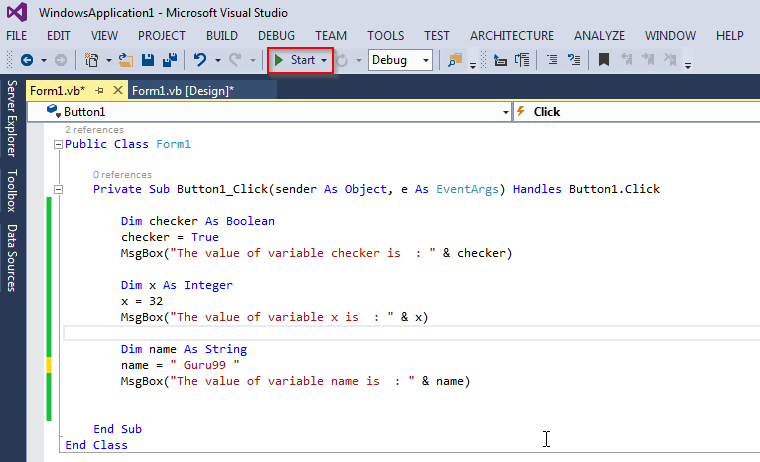Step 6) You should get the following form: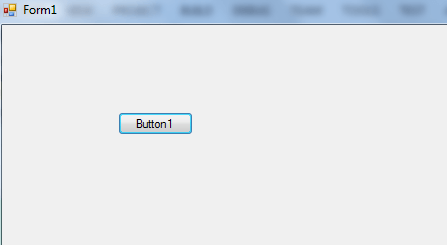Step 7) Click Button 1. You should get the following dialog: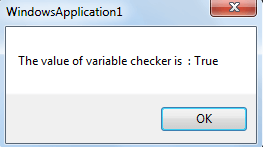Click the OK button to move to the next dialog. It should be as follows: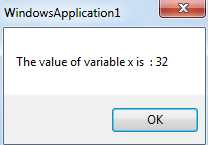Again, click the OK button to move to the next dialog. It should be as follows: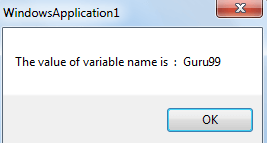Here is a screenshot of the complete code for the above: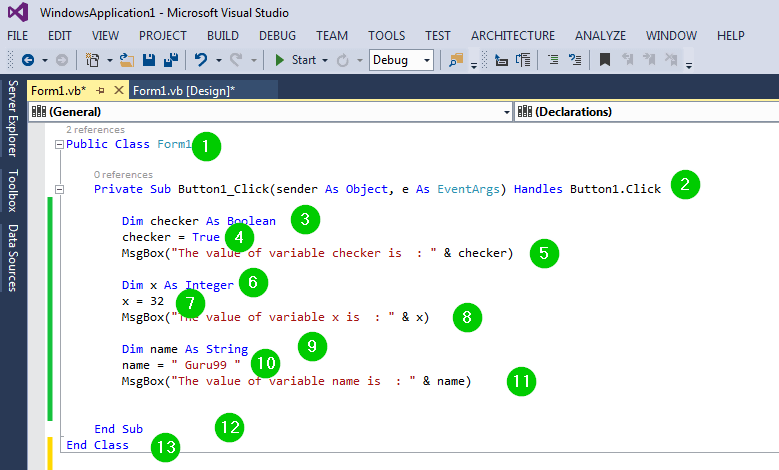Explanation of code:

1. Creating a public class named Form1
2. Creating a sub procedure named Button1_Click. It will be called when the button is clicked. The sender object will raise the event while the e argument will have data for the event. EventArgs forms the base class for all VB.Net event arguments. The Handles Button1.Click states that the subprocedure will handle any click on the button.
3. Creating a Boolean variable named checker.
4. Assigning a value of True to the checker variable.
5. Creating a Message Box dialog to show some text and the value of variable checker.
6. Creating an integer variable named x.
7. Assigning a value of 32 to the variable x.
8. Creating a Message Box dialog to show some text and the value of variable x.
9. Creating a string variable named name.
10. Assigning a variable of Guru99 to the variable name.
11. Creating a Message Box dialog to show some text and the value of variable name.
12. Ending the sub-procedure.
13. Ending the class.

## Accepting User Values

When creating an application, you may need a way of getting input from the user. This can be done using the ReadLine function of the Console class in System namespace. Once you have received the input from the user, you are required to assign it to a variable. For example:

```Dim user_message As String
```

In the above example, we have defined a variable named user_message. The message read from the console has been assigned to that variable. Let us demonstrate this:

Step 1) Create a Console Application

1. Open Visual Studio and click the File menu, Choose New then Project from the toolbar.2. On the new window, click Visual Basic from the left vertical navigation pane. Choose Console Application.1. Give it a name and click the OK button. The Project will be created.

Step 2) Add the following code to the workspace:

```Module Module1

Sub Main()
Dim user_message As String
Console.Write("Enter your message: ")
Console.WriteLine()
Console.WriteLine("You typed: {0}", user_message)
End Sub

End Module
```

Step 3) You can now run the code by clicking the Start button located at the top bar: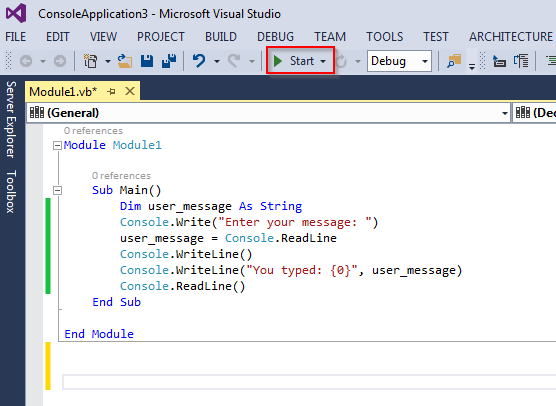Step 4) Enter your Message

1. On running the code, you will get the following console:1. Type in any message and hit the enter key. You should get the following: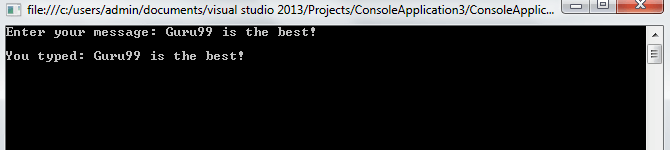Here is the complete code for the example: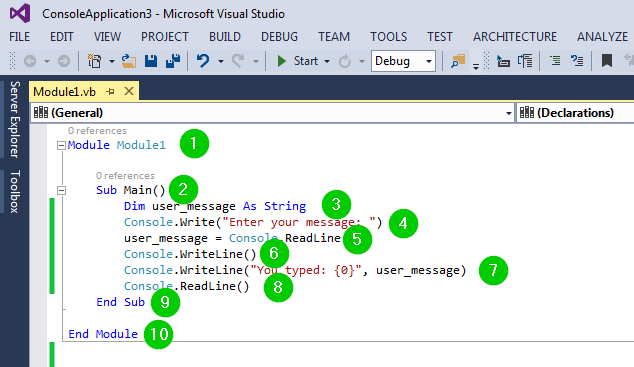Explanation of Code:

1. Creating a module named Module1. It will act as the class for the code.
2. Creating the main sub-procedure for the module. It will be invoked when the class is run/executed.
3. Creating a string variable named user_message.
4. Print a message on the console asking the user to type his/her message.
5. Assign the value read from the console to the above variable.
6. Printing an empty line on the console.
7. Print some text and the message typed by the user on the console.
8. Reading the message typed by the user.
9. End the sub-procedure.
10. End the module.

## Lvalues and Rvalues

VB.NET expressions are of two types:

• lvalue − an lvalue expression may appear on the left-hand or on the right-hand side of the assignment operator.
• rvalue – an rvalue expression can only appear on the right-hand of the assignment operator but not on the left-hand side.

Variables are lvalues, meaning that we can put them on the left side of the assignment operator. For example:

```Dim x As Integer = 32
```

For numeric literals, they can neither be assigned nor can they appear on the left-hand side of the assignment operators since they are rvalues. For example:

```32 = x
```

The above expression is wrong and will generate a compile-time error.

## Summary

• Each variable must belong to a data type. The data type determines the amount of memory space allocated to the variable.
• We can convert a variable from one data type to another.
• Initializing variables means assigning values to the variables.
• We create a console application to help us get input from the users via the console using the ReadLine function.